# High School Physics : Understanding Conservation of Energy

## Example Questions

### Example Question #1 : Energy And Work

A skier starts at the top of a hill with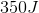of energy. Assuming energy is conserved, what is her final kinetic energy?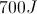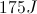Insufficient information to solve.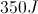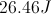Explanation:

If energy is conserved, then the total energy at the beginning equals the total energy at the end.

Since we have ONLY potential energy at the beginning and ONLY kinetic energy at the end,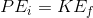.

Therefore, since our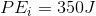, our kinetic energy will also equal.

### Example Question #1 : Energy And Work

A skier starts at the top of a hill with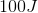of potential energy. At the bottom of the hill, she has only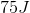of kinetic energy. Assuming that at the top of the hill she has only potential energy and at the bottom she has only kinetic energy, what can we conclude?

Work must have been done

The skier is at the bottom of one hill, but will go back up another

The skier must have paused somewhere during her descent

The skier is not a very good skier

The skier miscalculated her energies

Work must have been done

Explanation:

The work-energy theorem states that work is equal to change in energy, or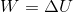.

Total mechanical energy is the sum of potential and kinetic energies: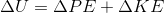In this case, she starts withand ends up with. Since there was a change of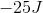, that means at some point during the system,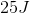of work was done by the skier.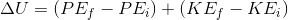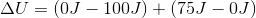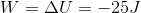### Example Question #3 : Understanding Conservation Of Energy

A rock is dropped in freefall from some initial height. Which of the following describes its final velocity right before it hits the ground?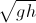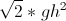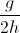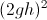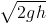Explanation:

For this problem, we must use the law of conservation of energy.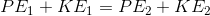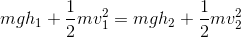Since the initial velocity is zero, there is no initial potential energy. Since the final height is zero, there is no final potential energy. This means that the final kinetic energy equals the initial potential energy.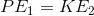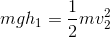The mass can be canceled from both sides.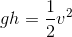Now we need to isolate the velocity by multiplying both sides by two, and taking the square root.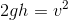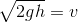### Example Question #1 : Understanding Conservation Of Energy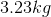book falls off the top of a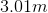bookshelf. What is its kinetic energy right before it hits the ground?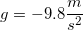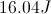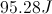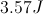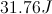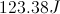Explanation:

Remember the law of conservation of energy: the total energy at the beginning equals the total energy at the end. In this case, we have only potential energy at the beginning and only kinetic energy at the end. (The initial velocity is zero, and the final height is zero).If we can find the potential energy, we can find the kinetic energy. The formula for potential energy is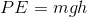.

Using our given values for the mass, height, and gravity, we can solve using multiplication. Note that the height becomes negative because the book is traveling in the downward direction.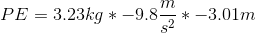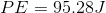The kinetic energy will also equal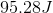, due to conservation of energy.

### Example Question #2 : Understanding Conservation Of Energy

A man stands on a tall ladder of height. He leans over a little too far and falls off the ladder. If his mass is, what is his kinetic energy right before he hits the ground?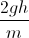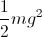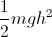Explanation:

For this problem, use the law of conservation of energy. This states that the total energy before the fall will equal the total energy after the fall. The initial kinetic energy will be zero, and the final potential energy will be zero; thus, the initial non-zero potential energy will be equal to the final non-zero kinetic energy.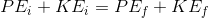We can use the energy equations to define these equal energies: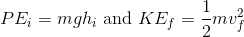The energies are equal, so we can say: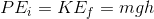### Example Question #6 : Understanding Conservation Of Energy

A skier waits at the top of a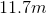hill. He then skis down the slope at an angle of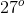above horizontal. What will his velocity be at the bottom of the hill?

We need to know the mass of the skier to solve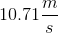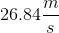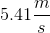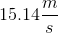Explanation:

To solve this problem, use the law of conservation of energy. The skier initially starts at rest; all of his initial energy will be potential energy. At the bottom of the hill, the potential energy will be zero and all of the final energy will be kinetic energy. We can set these two values equal to one another based on the conservation of energy principle.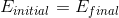Expand this equation to include the formulas for potential and kinetic energy.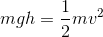Notice that the mass cancels out from both sides. This allows us to calculate without knowing the mass of the skier.Plug in our given values for the height of the slope and acceleration due to gravity. Since potential energy is a state function (independent of the path) the slope of the hill is irrelevant.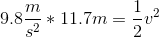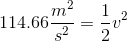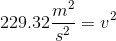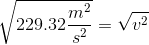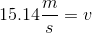### All High School Physics Resources